# Volume 19

## Rubik’s 4-Cube »NBCDFPDF

Published December 31, 2017

Rubiks cube has a natural extension to four-dimensional space. This article constructs the basic concepts of the puzzle and implements it in a program. The well-known three-dimensional Rubiks cube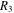consists of 27 unit subcubes. Each face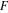ofdetermines a setof nine subcubes that have a face in the same plane as. The setcan be rotated around the normal through the center of. Rubiks 4-cube (or 4D hypercube)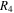consists of 81 unit 4-subcubes, each containing eight 3D subcubes. Each 3-face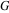ofdetermines a set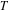of 27 4-subcubes that have a cube in the same hyperplane as. The setcan be rotated around the normal (a plane) through the center of. Projecting the whole 4D configuration to 3D exhibits Rubiks 4-cube as a four-dimensional extension of Rubiks cube. Starting from a random coloring of the 4-cube, the goal of the puzzle is to return to the initial coloring of the 3-faces. Read More »

## Every Clutter Is a Tree of Blobs »NBCDFPDF

Published December 4, 2017

Given a finite vertex set, one can construct every connected spanning hypergraph by first choosing a spanning hypertree, then choosing a blob on each of its edges. Read More »

## An Algorithm for Trigonometric-Logarithmic Definite Integrals »NBCDFPDF

Published October 20, 2017

We present a Mathematica implementation of an algorithm for computing new closed-form evaluations for classes of trig-logarithmic and hyperbolic-logarithmic definite integrals based on the substitution of logarithmic functions into the Maclaurin series expansions of trigonometric and hyperbolic functions. Using this algorithm, we offer new closed-form evaluations for a variety of trig-logarithmic integrals that state-of-the-art computer algebra systems cannot evaluate directly. We also show how this algorithm may be used to evaluate interesting infinite series and products. Read More »

## Calculating RRKM Rate Constants from Vibrational Frequencies and Their Dynamic Interpretation »NBCDFPDF

Published September 26, 2017

RiceRampsbergerKasselMarcus (RRKM) theory calculates an energy-dependent microcanonical unimolecular rate constant for a chemical reaction from a sum and density of vibrational quantum states. This article demonstrates how to program the BeyerSwinehart direct count of the sum and density of states for harmonic oscillators, as well as the SteinRabinovitch extension for anharmonic oscillators. Microcanonical rate constants are calculated for the decomposition of vinyl cyanide (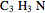) into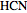,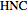and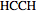as an example. Read More »

## Inversive Geometry »NBCDFPDF

### Part 3: Quandles, Inverting Triangles to Triangles and Inverting into Concentric Circles

Published August 24, 2017

This article continues the presentation of a variety of applications around the theme of inversion: quandles, inversion of one circle into another and inverting a pair of circles into congruent or concentric circles. Read More »

## Aspects of Input Shaping Control of Flexible Mechanical Systems »NBCDFPDF

Published June 15, 2017

Input shaping is an established technique to generate prefilters so that flexible mechanical systems move with minimal residual vibration. Many examples of such systems are found in engineeringfor example, space structures, robots, cranes and so on. The problem of vibration control is serious when precise motion is required in the presence of structural flexibility. In a wind turbine blade, untreated flapwise vibrations may reduce the life of the blade and unexpected vibrations can spread to the supporting structure. This article investigates one of the tools available to control vibrations within flexible mechanical systems using the input shaping technique. Read More »

## Polynomial L2 Approximation »NBCDFPDF

### Relating Orthonormal Polynomials, Gram—Schmidt Orthonormalization, QR Factorization, Normal Equations and Vandermonde and Hilbert Matrices

Published May 17, 2017

This didactic synthesis compares three solution methods for polynomial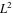approximation and systematically presents their common characteristics and their close interrelations:

1. Classical GramSchmidt orthonormalization and Fourier approximation in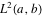2. Linear least-squares solution via QR factorization on an equally spaced grid in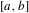3. Linear least-squares solution via the normal equations method inand on an equally
spaced grid inThe first two methods are linear least-squares systems with Vandermonde matrices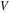; the normal equations contain matrices of Hilbert type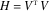. The solutions on equally spaced grids inconverge to the solutions in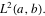All solution characteristics and their relations are illustrated by symbolic or numeric examples and graphs. Read More »

## Scattering and Gradient Forces from the Electromagnetic Stress Tensor Acting on a Dielectric Sphere »NBCDFPDF

Published March 28, 2017

The derivation of the scattering force and the gradient force on a spherical particle due to an electromagnetic wave often invokes the ClausiusMossotti factor, based on an ad hoc physical model. In this article, we derive the expressions including the ClausiusMossotti factor directly from the fundamental equations of classical electromagnetism. Starting from an analytic expression for the force on a spherical particle in a vacuum using the Maxwell stress tensor, as well as the Mie solution for the response of dielectric particles to an electromagnetic plane wave, we derive the scattering and gradient forces. In both cases, the ClausiusMossotti factor arises rigorously from the derivation without any physical argumentation. The limits agree with expressions in the literature. Read More »